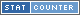# Calculate: 2 * 3 / 6 = 1

What is the answer for 2 * 3 / 6? Answer: 1

## How do you solve 2 * 3 / 6?

• Step #1 [Multiply] 2 * 3 = 6
• Step #2 [Divide] 6 / 6 = 1

## Word Phrase for 2 * 3 / 6 = 1

Internationalization (i18n) word phrase of the math problem 2 * 3 / 6 = 1

• English (EN): two multiply three divide six equals one
• Spanish (ES): dos multiplicar tres divisoria seis es igual a uno
• French (FR): deux multiplier trois diviser six ├Еgaux un
• German (DE): zwei multiplizieren drei teilen sechs ist gleich eins
• Italian (IT): due moltiplicarsi tre dividere sei uguale uno
• Hebrew (HE): ОЕОаОЎОЎОЮ ОюОћОЏОцОЎОю ОЕОюОЋОЕОћ ОћОцОеОЊ ОЕОЎОЕОћ ОЕОЋОЋОћ ОљОЌОЊ
• Indonesian (ID): dua bertambah banyak tiga membagi enam sama satu
• Russian (RU): СРЯ ЛЃл╝лйлЙлХлИЛѓЛї Ы­У Лђл░лил┤лхл╗лхлйлИлх ЭтыЫЧ Лђл░л▓лйлЙ ЬСУь
• Swedish (SV): tvт multiplicera tre klyftangens sex lika ett
• Turkish (TR): ├Дarpmak b├Хlmek e┼Ъittir

Q: Is the solution a whole number?
A: Yes, 1 is a whole number.

Q: Is the answer a positive or negative number?
A:The answer 1 is a positive number.

## Solve in Base Systems

The equation 2 * 3 / 6 = 1 is represented in base 10 above. Here we show the same calculation but represented in other base counting systems. A base counting system is how many numbers are represented as group before advancing to the next digit. Example we normally use base 10 with numbers 0 to 9. When we add a 1 to the number 9 it becomes 10. For a base 3 system when a 1 is added to 2, it does not become 3 it becomes 10.

 Base Base Equation Base Answer 2 (binary) 10 * 11 / 110 1 3 2 * 10 / 20 1 4 2 * 3 / 12 1 5 2 * 3 / 11 1 6 2 * 3 / 10 1 7 2 * 3 / 6 1

## Simular problems to 2 * 3 / 6 = 1